# Current Electricity Current Electricity what have we discussed

• Slides: 76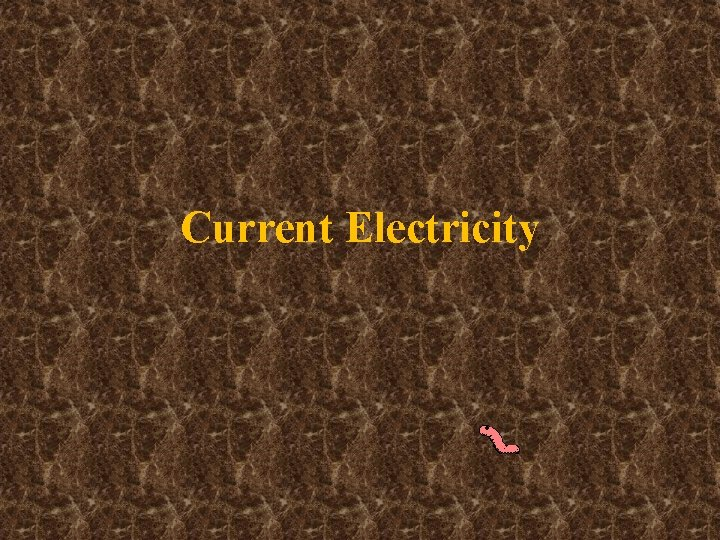Current Electricity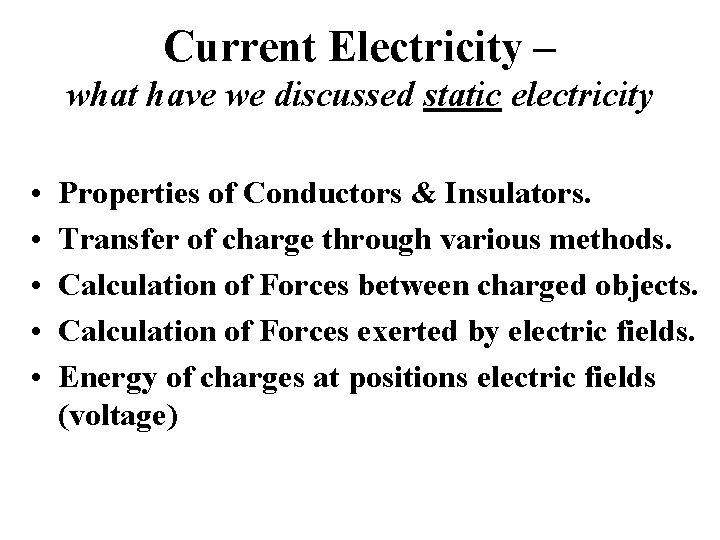Current Electricity – what have we discussed static electricity • • • Properties of Conductors & Insulators. Transfer of charge through various methods. Calculation of Forces between charged objects. Calculation of Forces exerted by electric fields. Energy of charges at positions electric fields (voltage)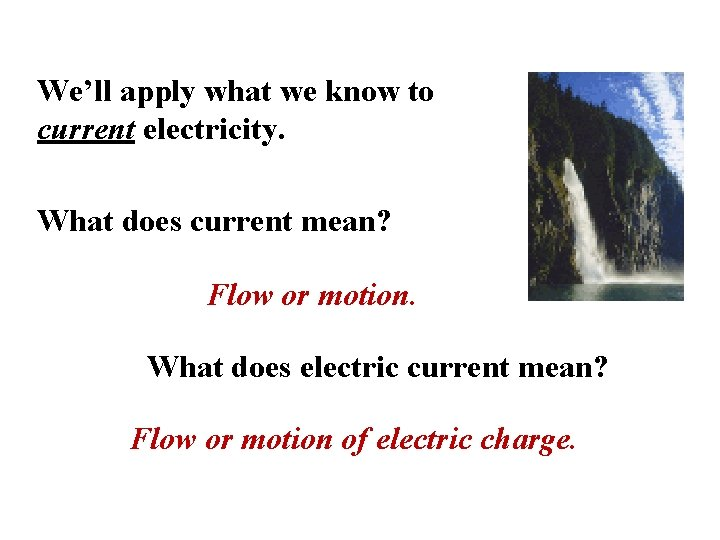We’ll apply what we know to current electricity. What does current mean? Flow or motion. What does electric current mean? Flow or motion of electric charge.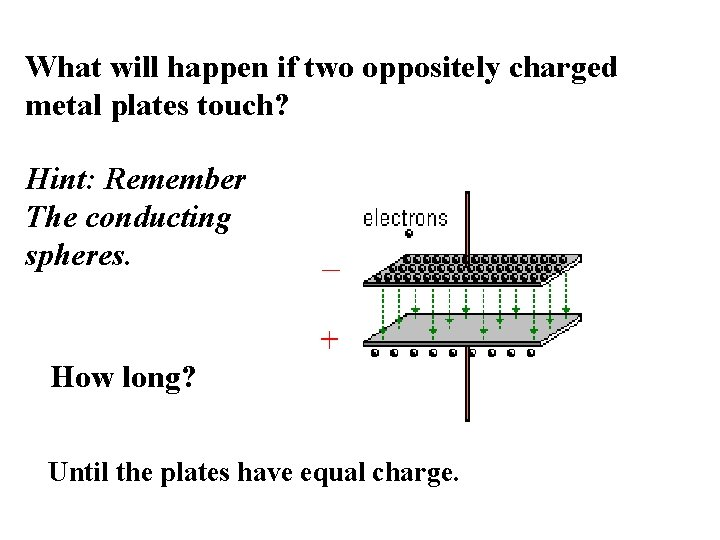What will happen if two oppositely charged metal plates touch? Hint: Remember The conducting spheres. How long? Until the plates have equal charge.We can use E of charges in motion to do work. If we transfer charges from high PE to low PE, the charge can do some work converting E– but not for long.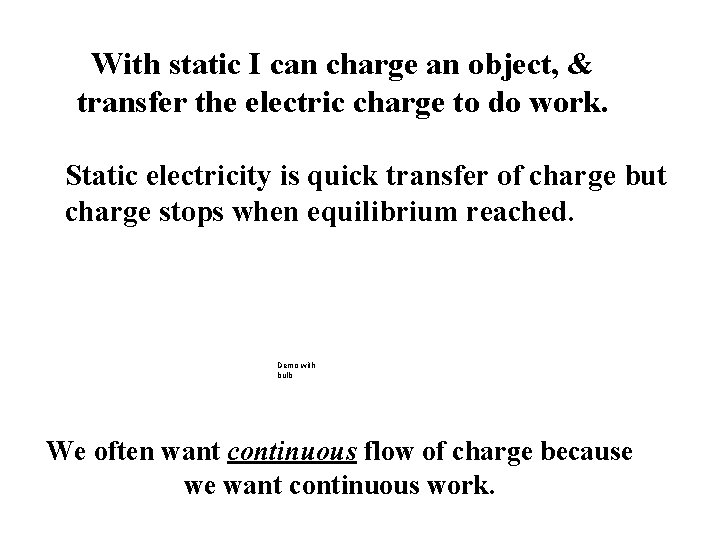With static I can charge an object, & transfer the electric charge to do work. Static electricity is quick transfer of charge but charge stops when equilibrium reached. Demo with bulb We often want continuous flow of charge because we want continuous work.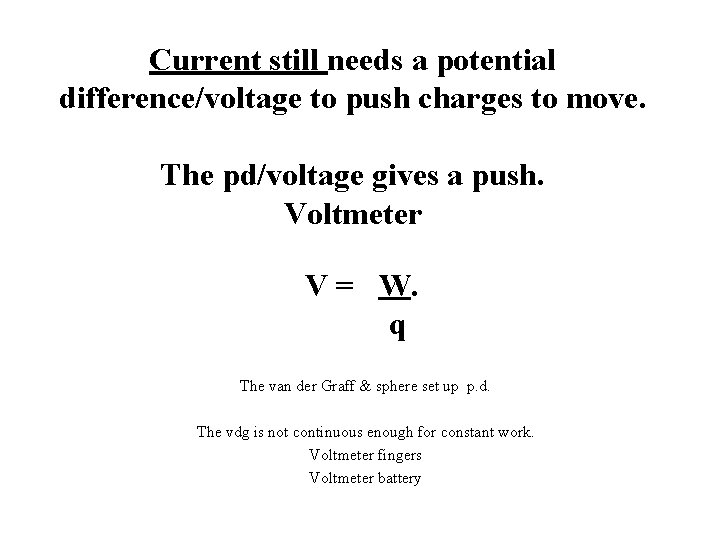Current still needs a potential difference/voltage to push charges to move. The pd/voltage gives a push. Voltmeter V = W. q The van der Graff & sphere set up p. d. The vdg is not continuous enough for constant work. Voltmeter fingers Voltmeter battery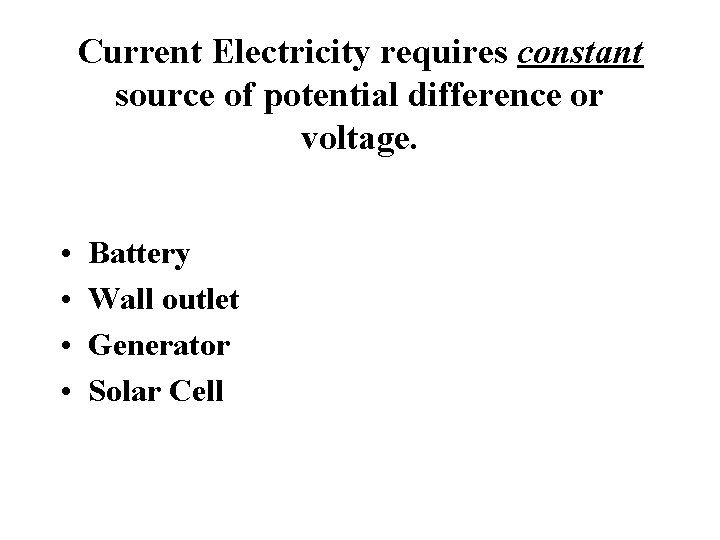Current Electricity requires constant source of potential difference or voltage. • • Battery Wall outlet Generator Solar Cell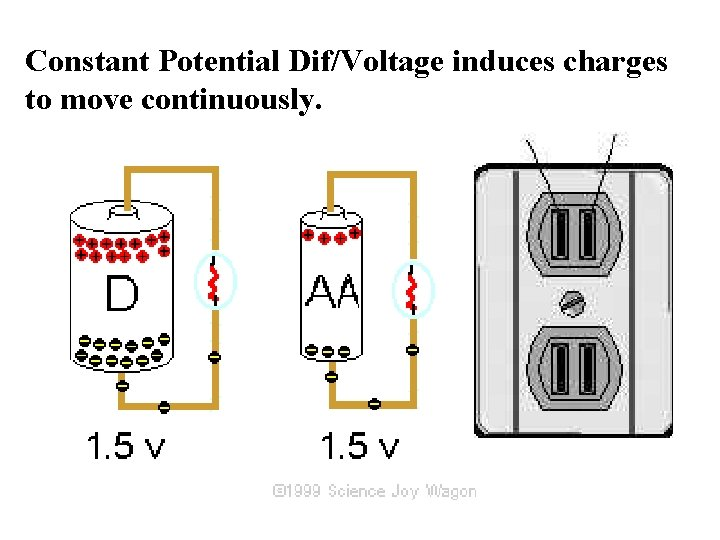Constant Potential Dif/Voltage induces charges to move continuously.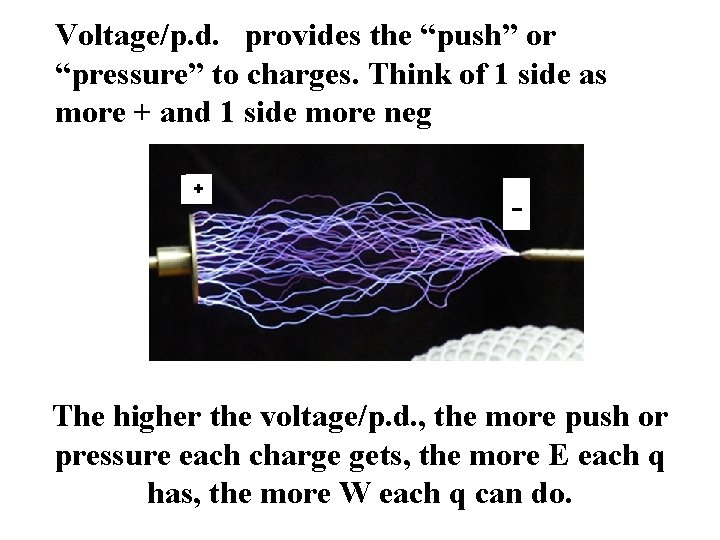Voltage/p. d. provides the “push” or “pressure” to charges. Think of 1 side as more + and 1 side more neg + - The higher the voltage/p. d. , the more push or pressure each charge gets, the more E each q has, the more W each q can do.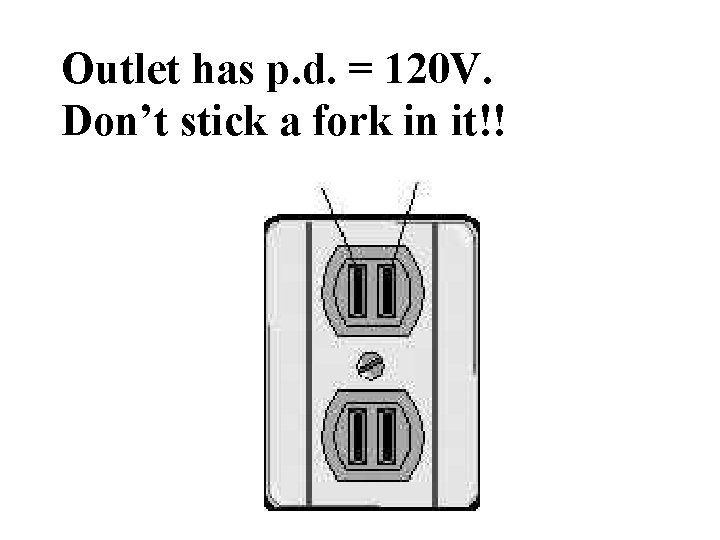Outlet has p. d. = 120 V. Don’t stick a fork in it!!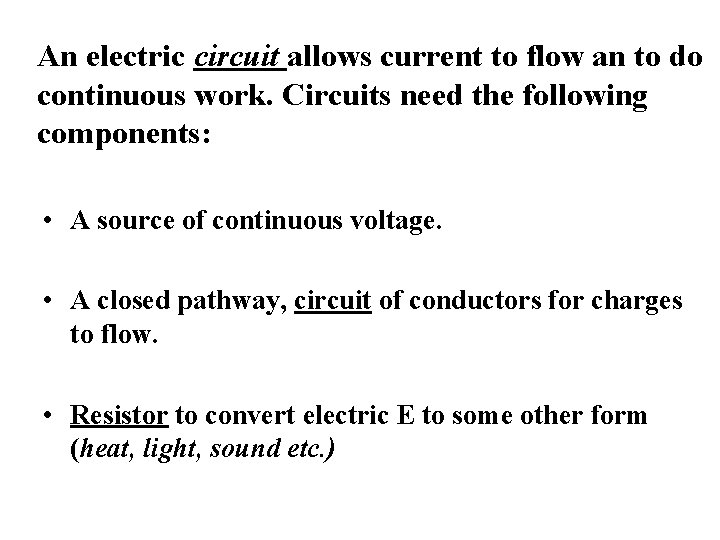An electric circuit allows current to flow an to do continuous work. Circuits need the following components: • A source of continuous voltage. • A closed pathway, circuit of conductors for charges to flow. • Resistor to convert electric E to some other form (heat, light, sound etc. )Which type of charges can move? Solids only e- flow. Liquids any charged particles or ions can flow.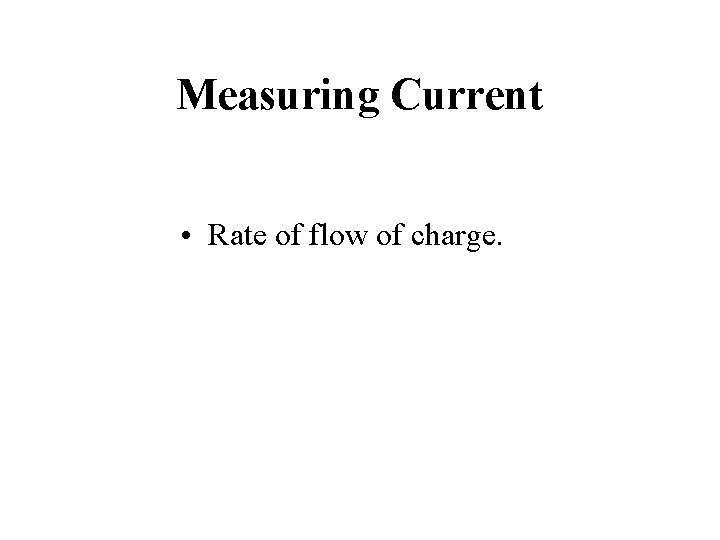Measuring Current • Rate of flow of charge.# charges per sec or # Coulombs per sec. Amperes (A) measures rate of coulombs passing a point in a wire. 1 A = 1 C/s passing a point or cross section of wire.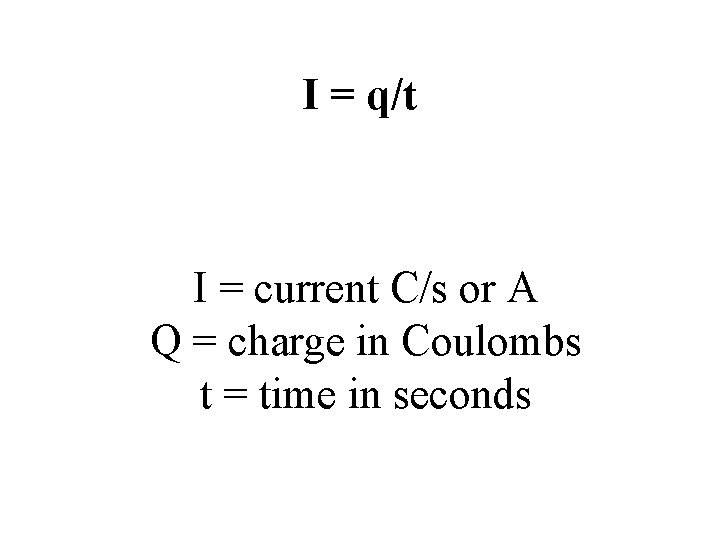I = q/t I = current C/s or A Q = charge in Coulombs t = time in seconds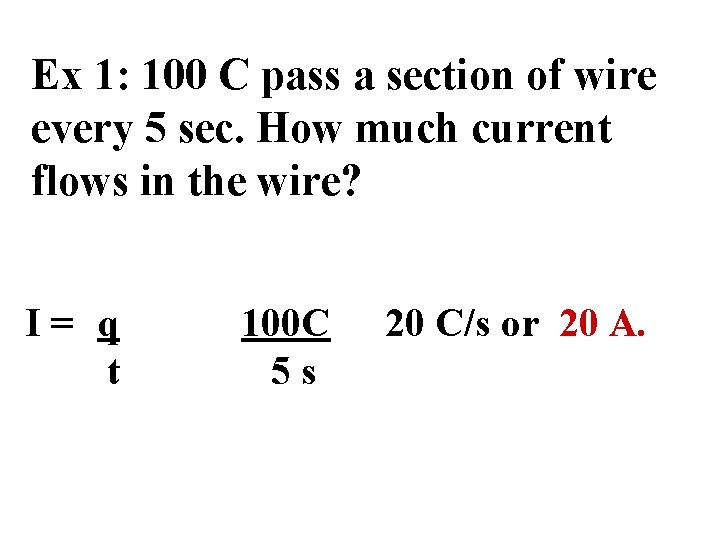Ex 1: 100 C pass a section of wire every 5 sec. How much current flows in the wire? I= q t 100 C 5 s 20 C/s or 20 A.Ex 2: The current in a light bulb is 0. 835 A. How long does it take for a total charge of 1. 67 C to pass a point in the wire? I = Q/t t = 1. 67 C 0. 835 C/s t = Q/I = 2. 00 s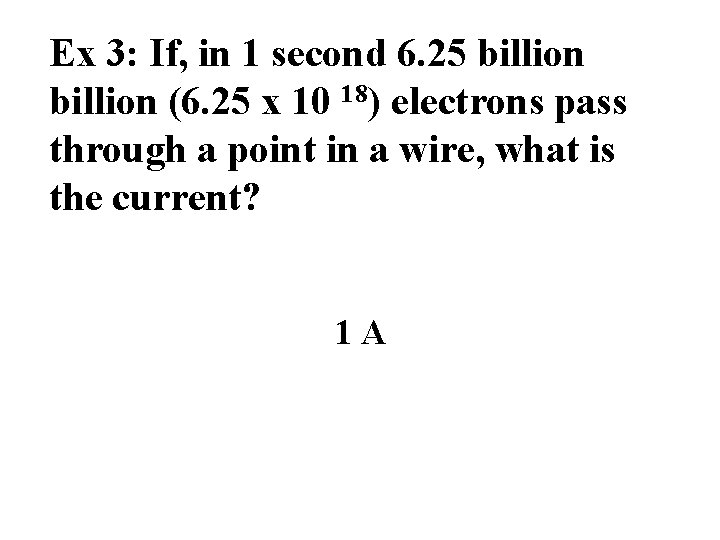Ex 3: If, in 1 second 6. 25 billion (6. 25 x 10 18) electrons pass through a point in a wire, what is the current? 1 ATo get continuous flow of charge we need: 1. voltage (p. d. ) to push charges. 2. Charge Pump / E source = do work on charge which gains PE. Pumps are batteries, generators. 3. Closed Circuit – continuous pathway for charges to flow –metal wire.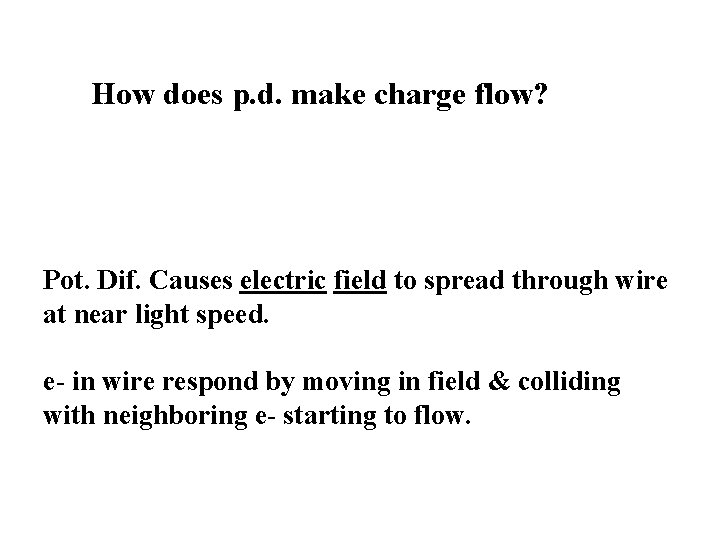How does p. d. make charge flow? Pot. Dif. Causes electric field to spread through wire at near light speed. e- in wire respond by moving in field & colliding with neighboring e- starting to flow.Electric field in wire caused by voltage source induces all e- to move. Each e- moves slowly but all begin at once.• Simple Elec Circuit 5. 5 min • https: //www. youtube. com/watch? v=EJe. Au. Q 7 pkpc&app=d esktop • Senior Physics Electric Current Clip 9 minutes • http: //www. youtube. com/watch? v=5 la. Tkj. INHrg • Senior Physics Electric Voltage (skiers) 10 minutes • http: //www. youtube. com/watch? v=F 1 p 3 fgb. Dnk. Y • Battery clip old but nice. 10 min. • http: //www. youtube. com/watch? v=Ipa. EGhjp. ZgcCircuitsCircuit – Closed pathway for charges. All components need p. d. to move. Potential Dif/ Voltage Gives the push Conducting path (wire). Easy to move current through very little p. d. needed. Resistors – (bulbs) Difficult for current to flow. Larger p. d. needed.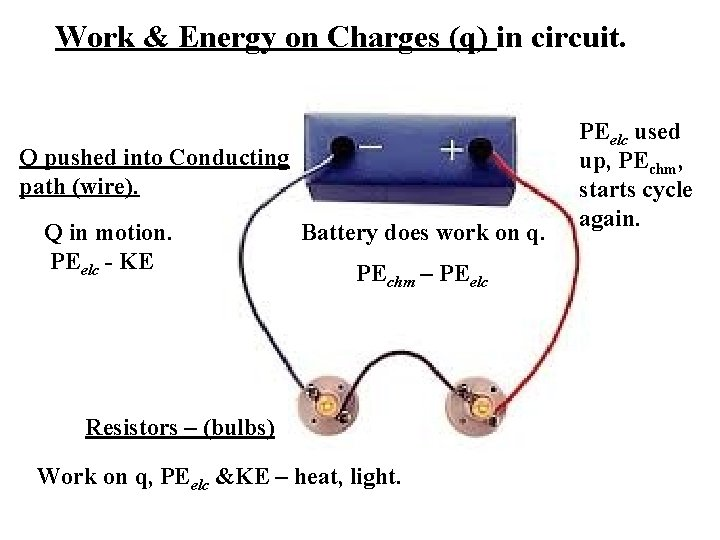Work & Energy on Charges (q) in circuit. Q pushed into Conducting path (wire). Q in motion. PEelc - KE Battery does work on q. PEchm – PEelc Resistors – (bulbs) Work on q, PEelc &KE – heat, light. PEelc used up, PEchm, starts cycle again.A Closer Look at Resistors Bulbs, toasters, computers. . . convert KE of e- to other forms of E – heat, light etc. Devices are called resistors or loads. They slow down the e- so they resist current flow. (Like paddle wheel in river).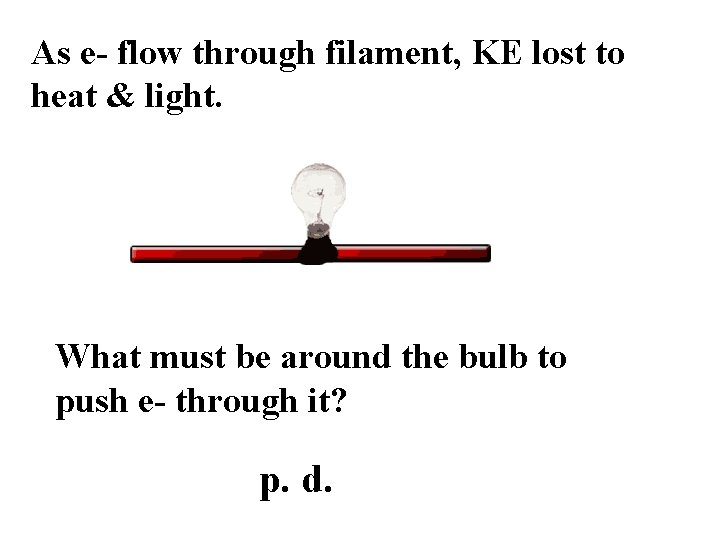As e- flow through filament, KE lost to heat & light. What must be around the bulb to push e- through it? p. d.Ohm’s Law Relates Resistance, Current, Voltage R = V/I V = volts J/C I = current A, C/s R = resistance ohm’s W or V/A. Resistance (R) unit = ohm’s W.1. A 120 -V potential difference is applied to a toaster which draws a current of 4 A. What is the toaster’s resistance? • R – V/I • = 120 -V/4 A • =30 W.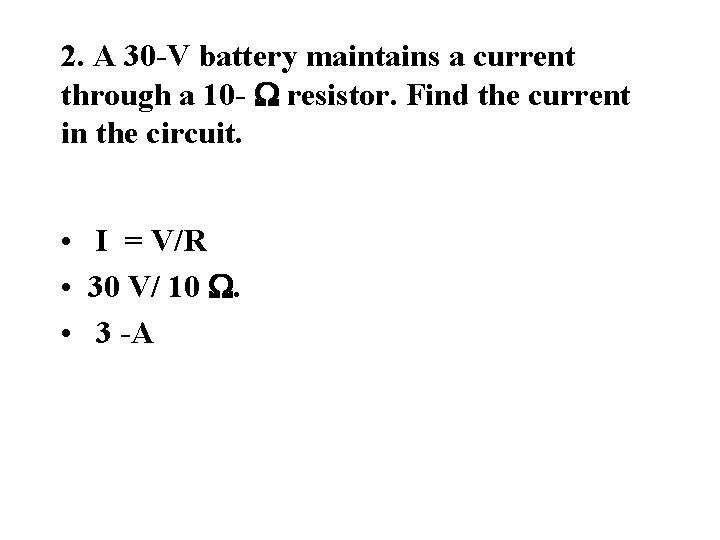2. A 30 -V battery maintains a current through a 10 - W resistor. Find the current in the circuit. • I = V/R • 30 V/ 10 W. • 3 -A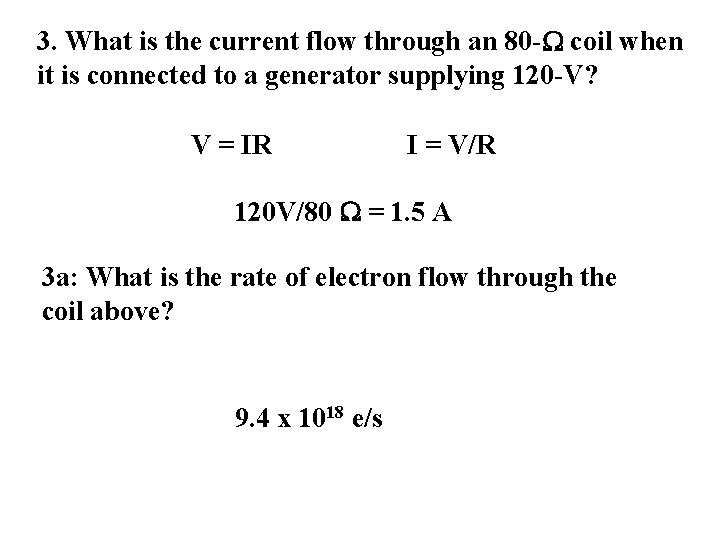3. What is the current flow through an 80 -W coil when it is connected to a generator supplying 120 -V? V = IR I = V/R 120 V/80 W = 1. 5 A 3 a: What is the rate of electron flow through the coil above? 9. 4 x 1018 e/s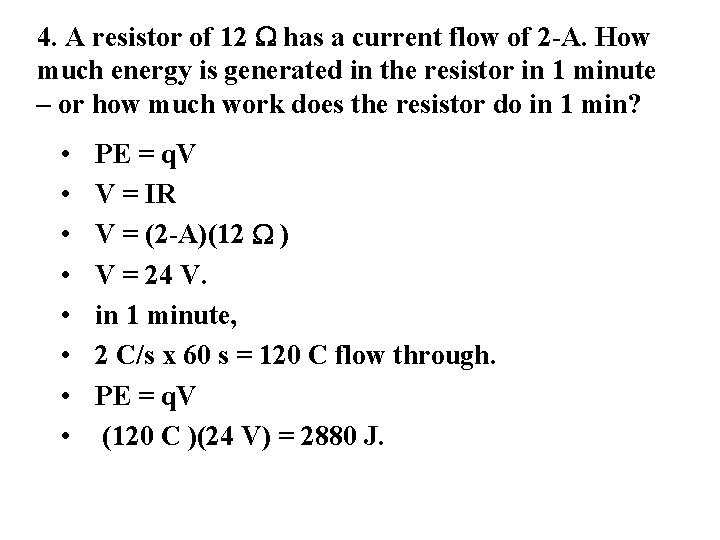4. A resistor of 12 W has a current flow of 2 -A. How much energy is generated in the resistor in 1 minute – or how much work does the resistor do in 1 min? • • PE = q. V V = IR V = (2 -A)(12 W ) V = 24 V. in 1 minute, 2 C/s x 60 s = 120 C flow through. PE = q. V (120 C )(24 V) = 2880 J.Hwk: Read Text 19 -1 and 19 -2. Do pg 695 #3 -5 and pg 703 # 3 -5.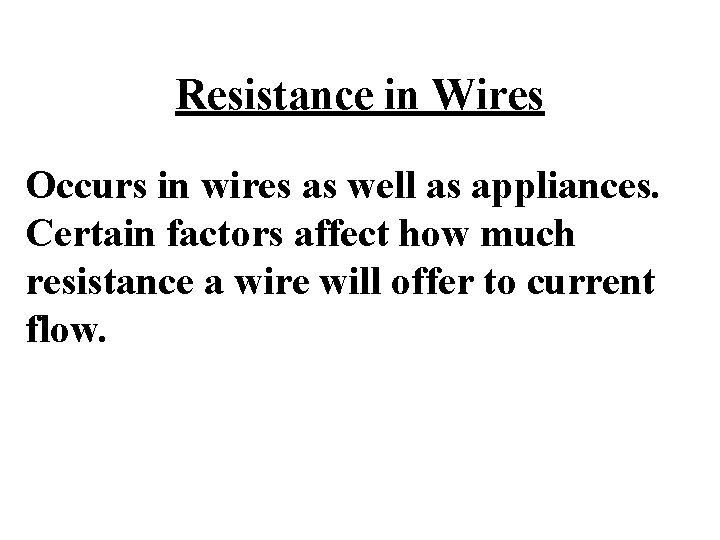Resistance in Wires Occurs in wires as well as appliances. Certain factors affect how much resistance a wire will offer to current flow.Factors affecting wire resistance. 1. Length 2. Area 3. Temperature 4. Type of material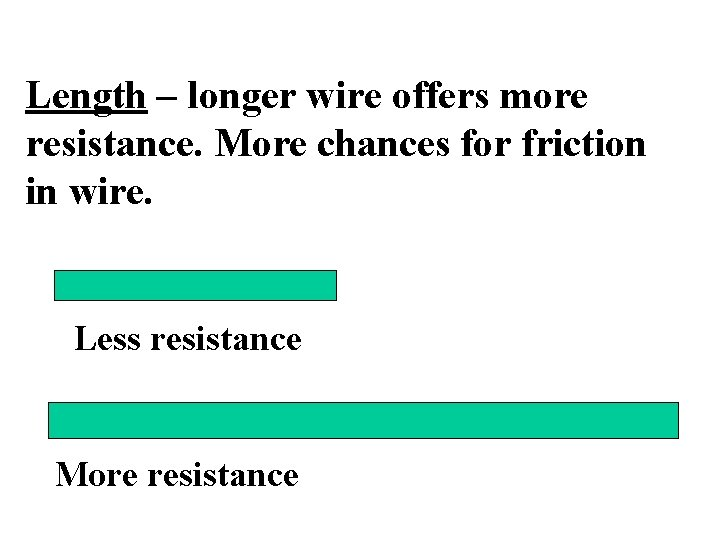Length – longer wire offers more resistance. More chances for friction in wire. Less resistance More resistanceCross Sectional Area Thick wires offer less resistance.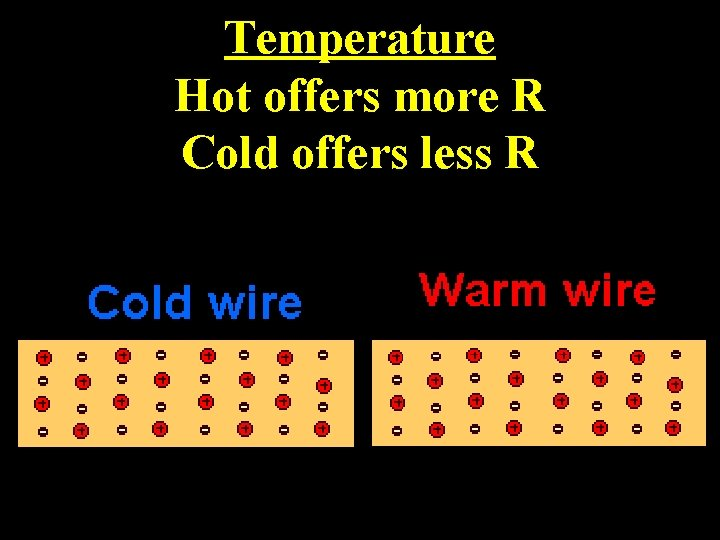Temperature Hot offers more R Cold offers less R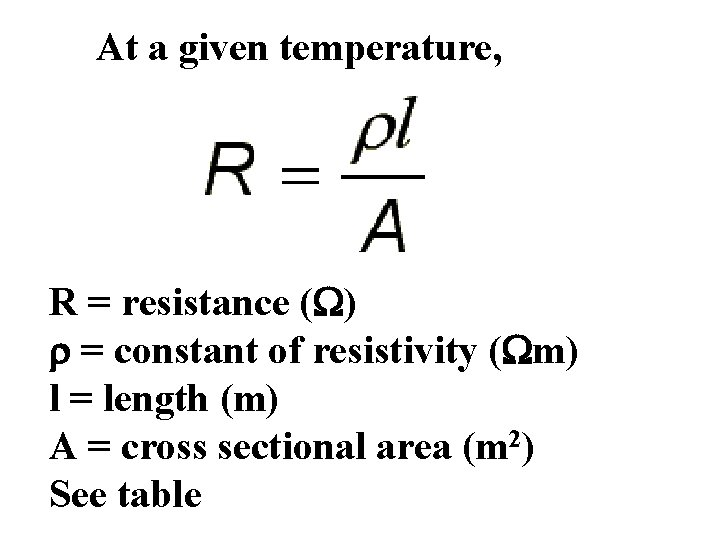At a given temperature, R = resistance (W) r = constant of resistivity (Wm) l = length (m) A = cross sectional area (m 2) See tableEx 1: A 9. 5 cm length of copper wire has a cross sectional area -3 2 of 2. 5 x 10 m. What is the o resistance of the wire at 20 C?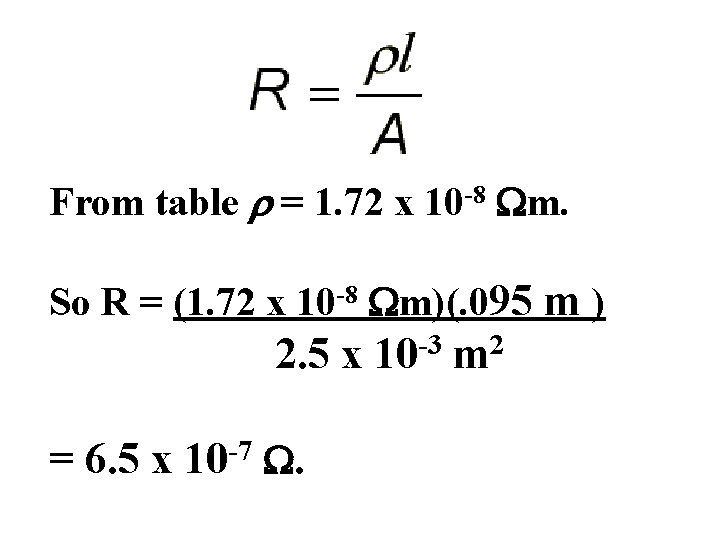From table r = 1. 72 x 10 -8 Wm. So R = (1. 72 x 10 -8 Wm)(. 095 m ) 2. 5 x 10 -3 m 2 = 6. 5 x 10 -7 W.Ex 2: Find the resistance of a copper wire 10 m long and 1. 2 x 10 -9 m 2 in area. 143 W.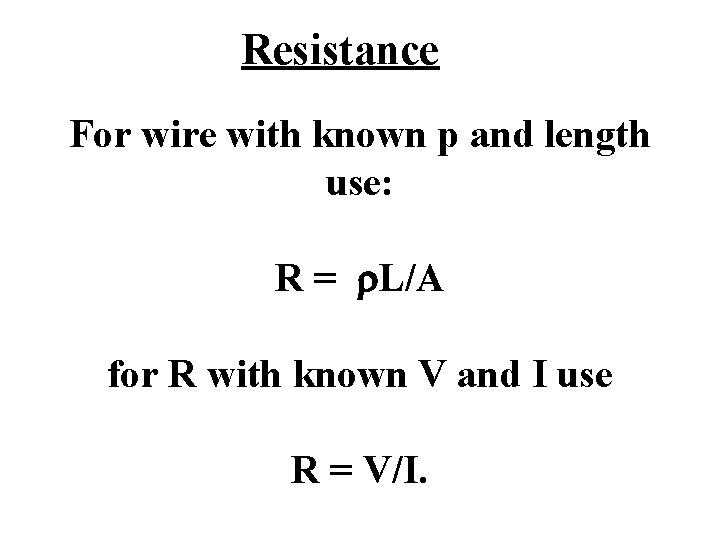Resistance For wire with known p and length use: R = r. L/A for R with known V and I use R = V/I.Film clip with questions. 9: 30 minutes. https: //www. youtube. com/watch? v=GYJa. Ovk. SEPw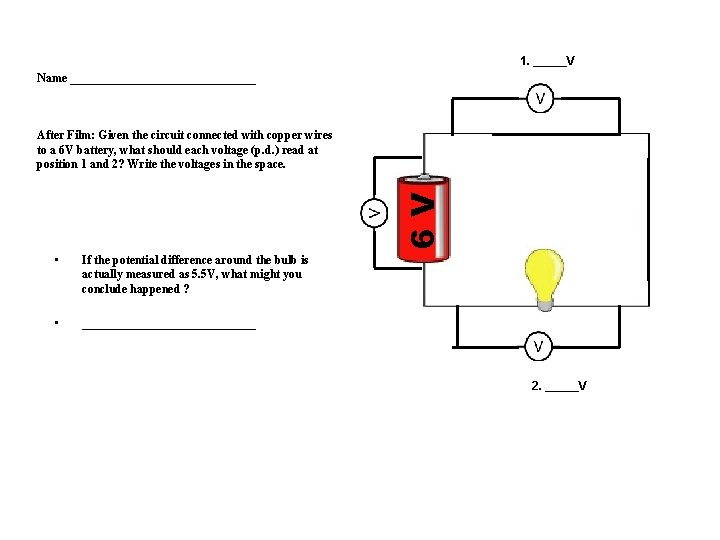1. _____V Name ________________ 6 V After Film: Given the circuit connected with copper wires to a 6 V battery, what should each voltage (p. d. ) read at position 1 and 2? Write the voltages in the space. • If the potential difference around the bulb is actually measured as 5. 5 V, what might you conclude happened ? • _______________ 2. _____V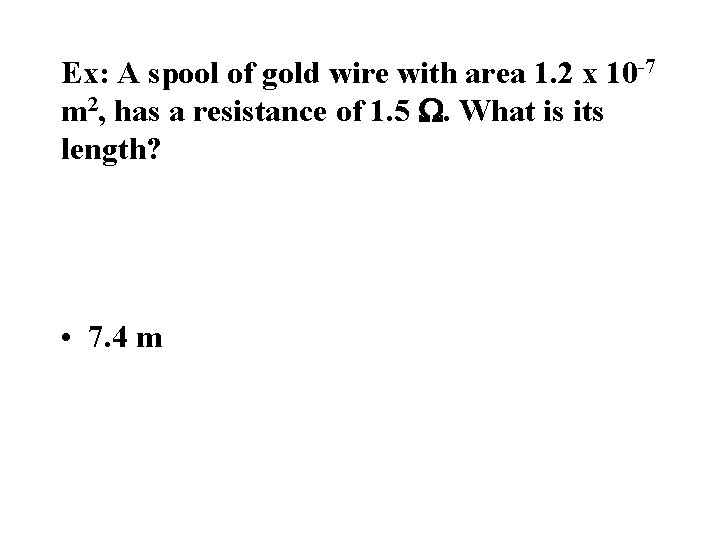Ex: A spool of gold wire with area 1. 2 x 10 -7 m 2, has a resistance of 1. 5 W. What is its length? • 7. 4 m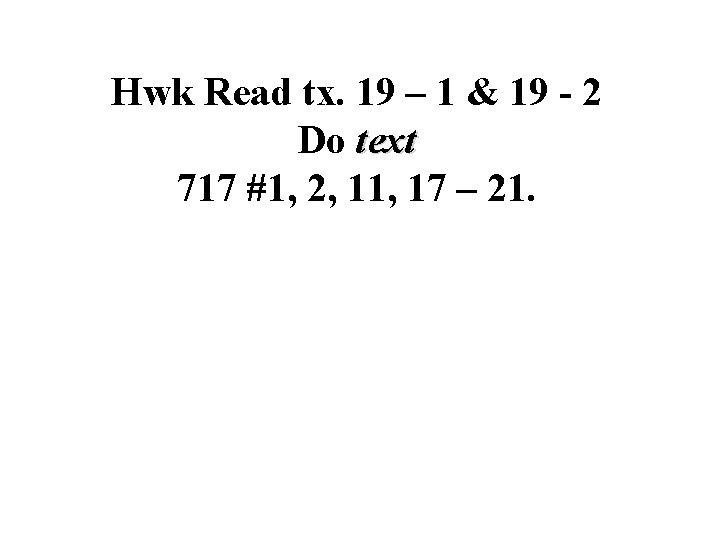Hwk Read tx. 19 – 1 & 19 - 2 Do text 717 #1, 2, 11, 17 – 21.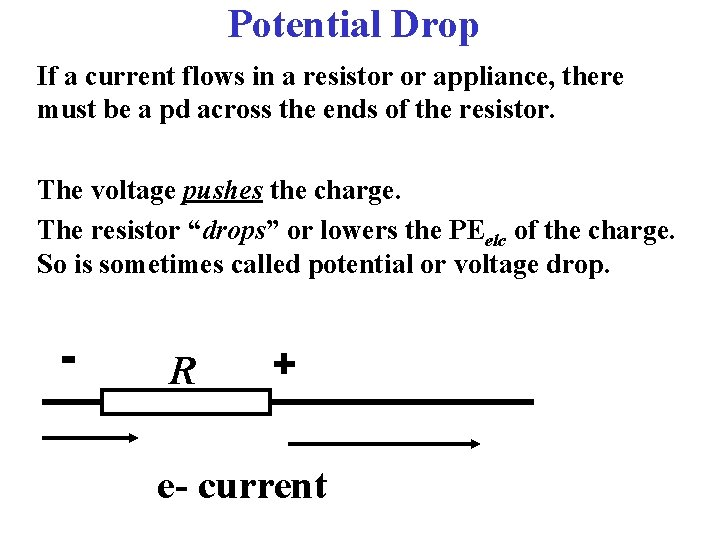Potential Drop If a current flows in a resistor or appliance, there must be a pd across the ends of the resistor. The voltage pushes the charge. The resistor “drops” or lowers the PEelc of the charge. So is sometimes called potential or voltage drop. - R + e- currentCurrent (flow rate) increases w/ increased pd & decreases with increased resistance. • R = V/I • I=V R Or units V/W V = IRWhat is the graph of Ohm’s Law? Usu V on y-axis, I on x-axis, R is slope of direct linear.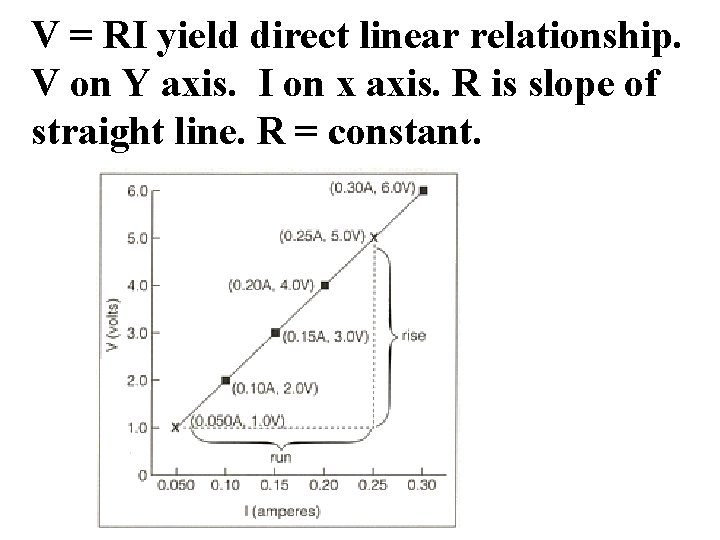V = RI yield direct linear relationship. V on Y axis. I on x axis. R is slope of straight line. R = constant.What would the slope represent if current was placed on the Y axis? 1/R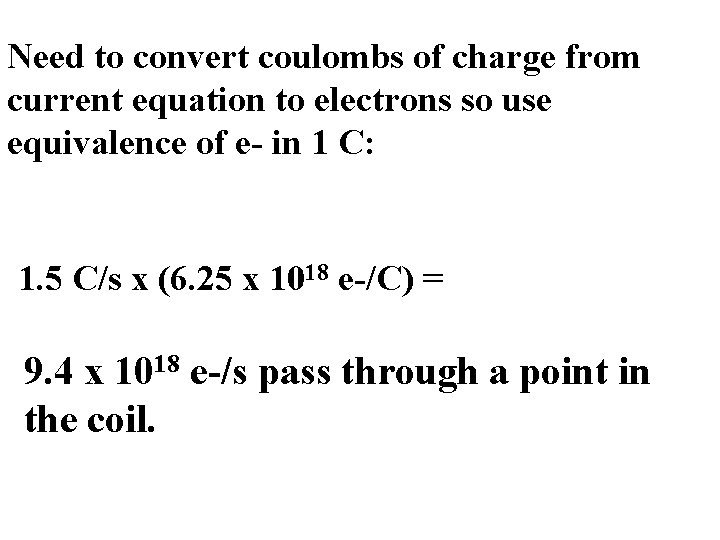Need to convert coulombs of charge from current equation to electrons so use equivalence of e- in 1 C: 1. 5 C/s x (6. 25 x 1018 e-/C) = 9. 4 x 1018 e-/s pass through a point in the coil.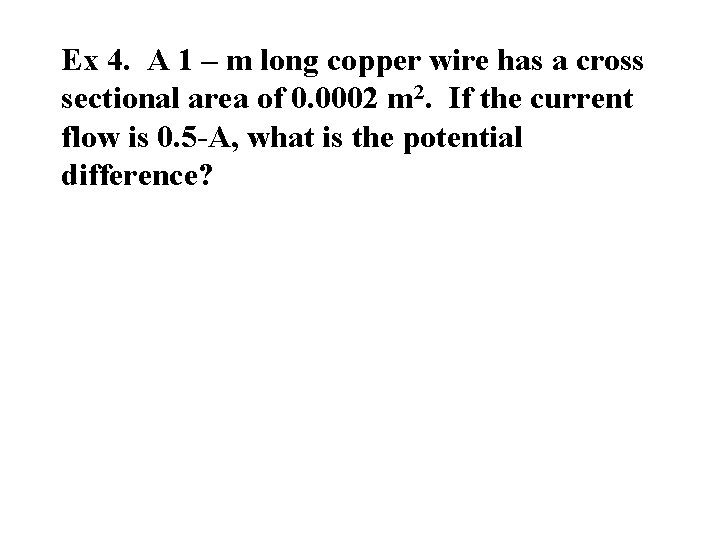Ex 4. A 1 – m long copper wire has a cross sectional area of 0. 0002 m 2. If the current flow is 0. 5 -A, what is the potential difference?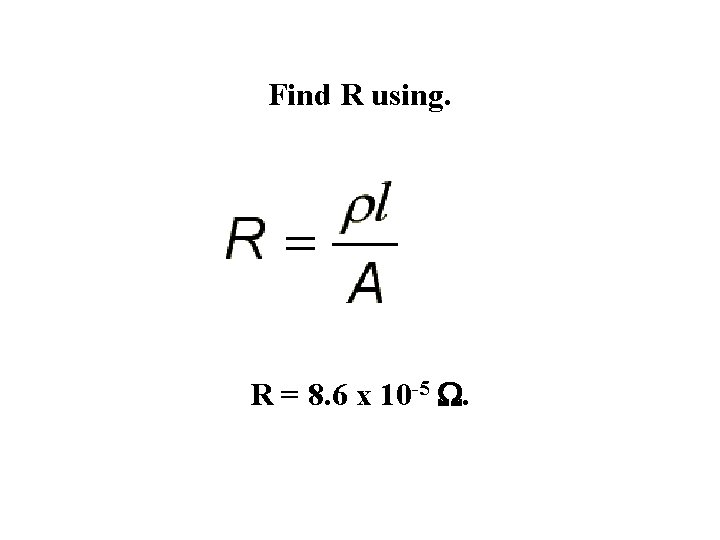Find R using. R = 8. 6 x 10 -5 W.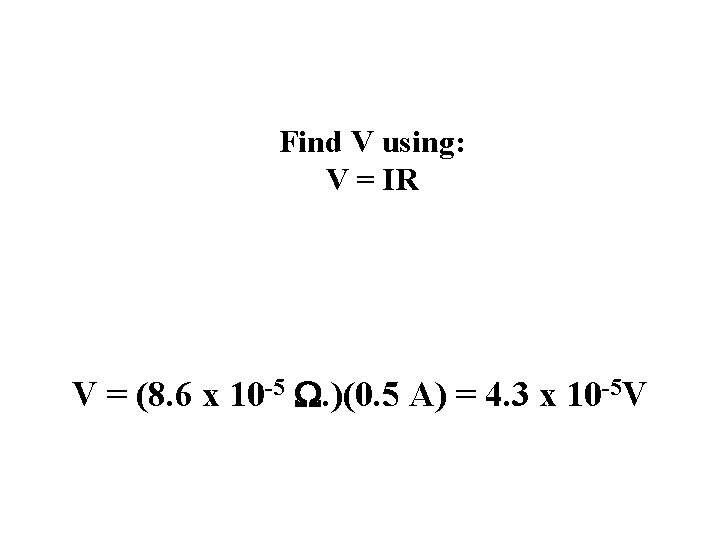Find V using: V = IR V = (8. 6 x 10 -5 W. )(0. 5 A) = 4. 3 x 10 -5 V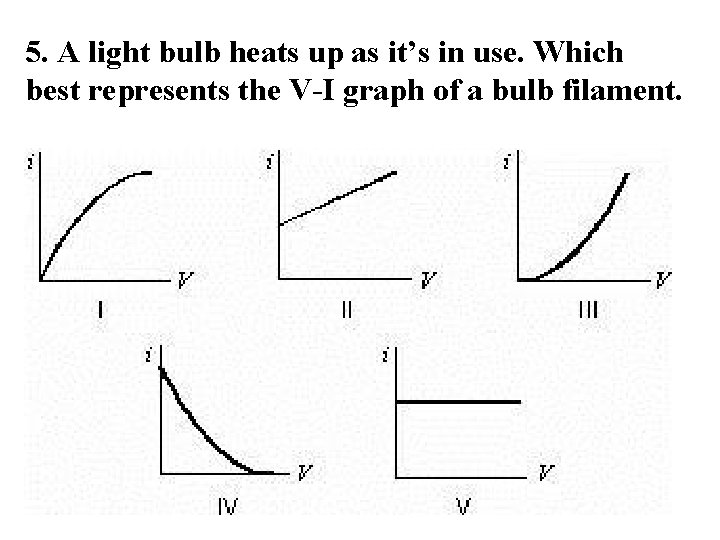5. A light bulb heats up as it’s in use. Which best represents the V-I graph of a bulb filament.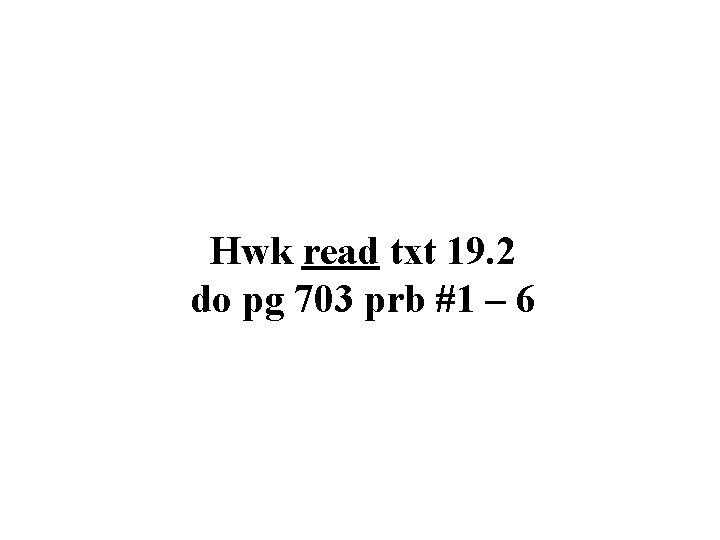Hwk read txt 19. 2 do pg 703 prb #1 – 6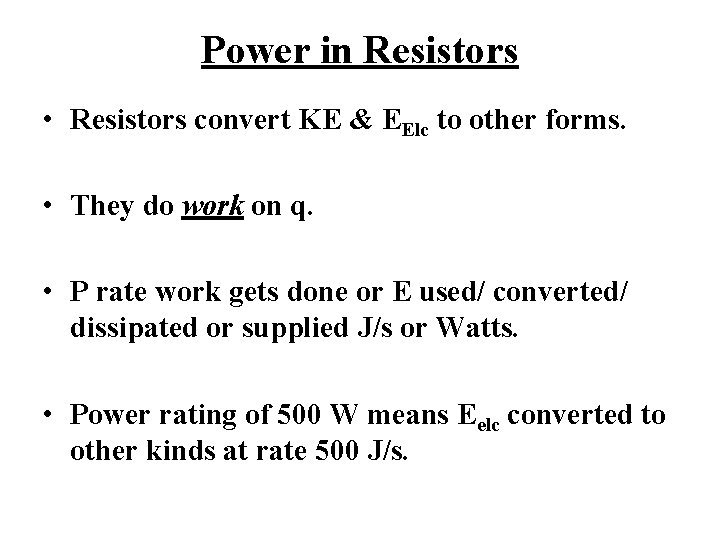Power in Resistors • Resistors convert KE & EElc to other forms. • They do work on q. • P rate work gets done or E used/ converted/ dissipated or supplied J/s or Watts. • Power rating of 500 W means Eelc converted to other kinds at rate 500 J/s.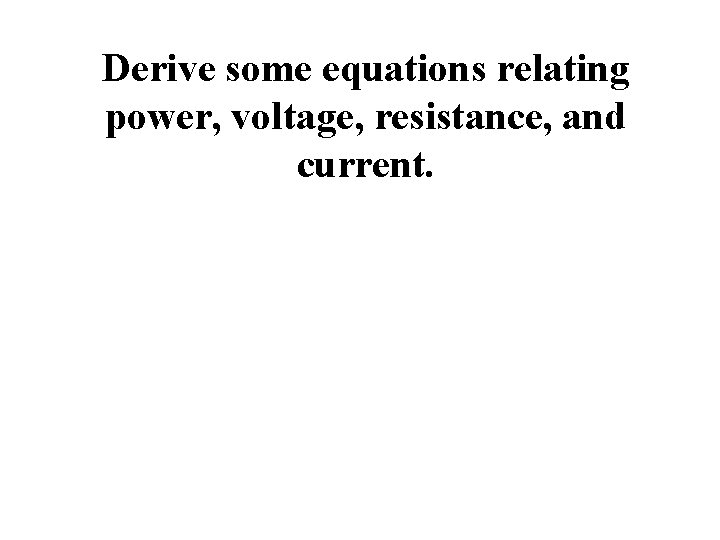Derive some equations relating power, voltage, resistance, and current.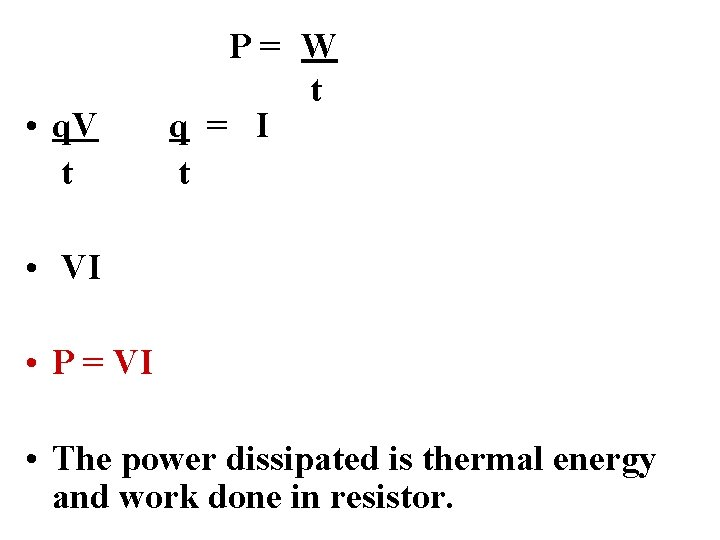• q. V t P= W t q = I t • VI • P = VI • The power dissipated is thermal energy and work done in resistor.Other Power Equations From Ohm’s Law we use R = V/I to derive other equations for power. P = I 2 R = V 2. REx 1. A toaster draws a current of 2. 0 -A from a 120 -V source. What is its power rating? P = VI P = (120 V)(2. 0 A) 240 VA or 240 W.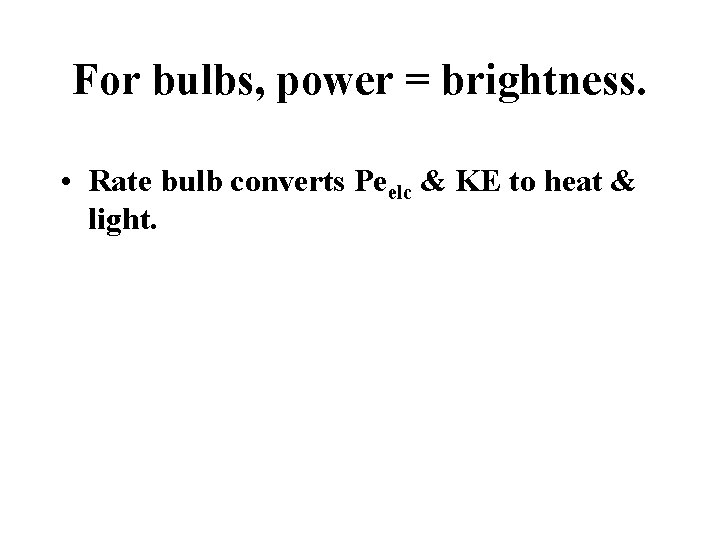For bulbs, power = brightness. • Rate bulb converts Peelc & KE to heat & light.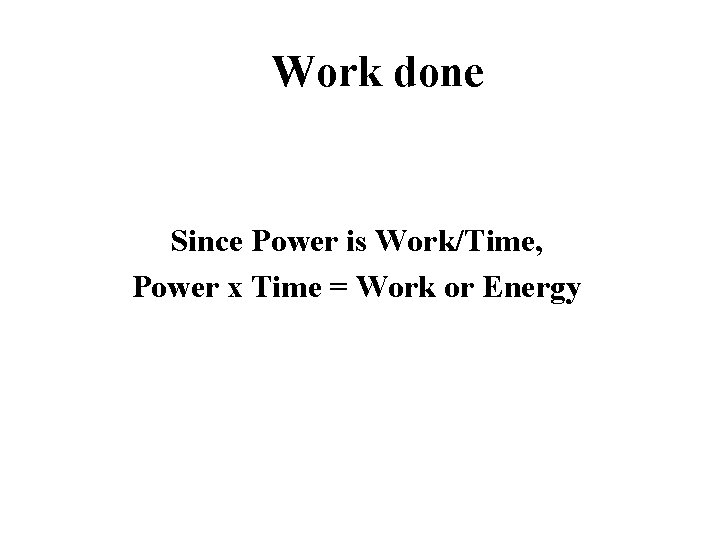Work done Since Power is Work/Time, Power x Time = Work or Energy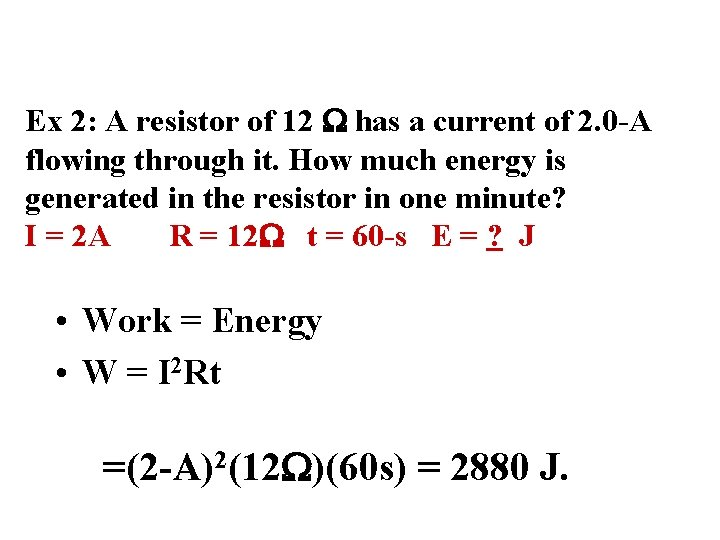Ex 2: A resistor of 12 W has a current of 2. 0 -A flowing through it. How much energy is generated in the resistor in one minute? I = 2 A R = 12 W t = 60 -s E = ? J • Work = Energy • W = I 2 Rt =(2 -A)2(12 W)(60 s) = 2880 J.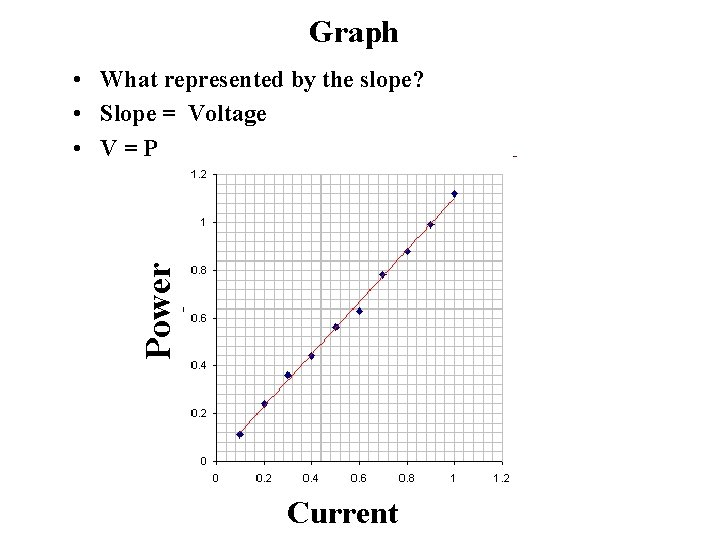Graph Power • What represented by the slope? • Slope = Voltage • V=P Current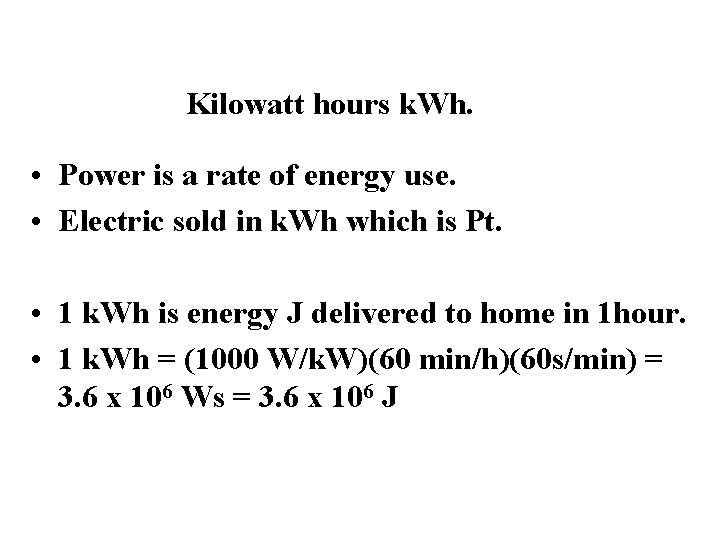Kilowatt hours k. Wh. • Power is a rate of energy use. • Electric sold in k. Wh which is Pt. • 1 k. Wh is energy J delivered to home in 1 hour. • 1 k. Wh = (1000 W/k. W)(60 min/h)(60 s/min) = 3. 6 x 106 Ws = 3. 6 x 106 JPower Ratings for Appliances Devices are rated by the power they use. A bulb rated 60 W 220 V means: the bulb will dissipate 60 W when attached to a 220 p. d. If a different p. d. is used, then it won’t dissipate 60 W.Fuses As current flows, wires heat up. Fuses designed to break circuit if current becomes to high for the wires. Fuse should be rated just above the ideal operating current for a circuit.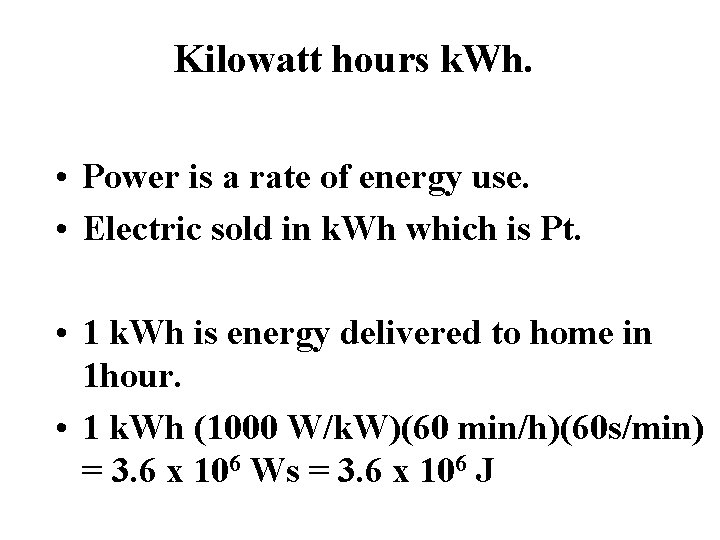Kilowatt hours k. Wh. • Power is a rate of energy use. • Electric sold in k. Wh which is Pt. • 1 k. Wh is energy delivered to home in 1 hour. • 1 k. Wh (1000 W/k. W)(60 min/h)(60 s/min) = 3. 6 x 106 Ws = 3. 6 x 106 J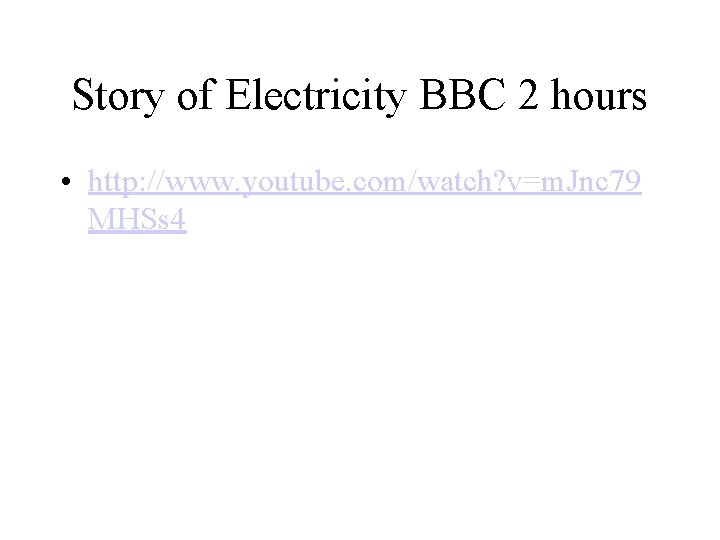Story of Electricity BBC 2 hours • http: //www. youtube. com/watch? v=m. Jnc 79 MHSs 4Finish Power Examples Hwk Read 19. 3 Text do p 710 1 – 4, p 713 #1 – 3 and finish prac set